# Introduction to Contact Modeling

Today I have the great pleasure to introduce my colleague Sebastian Castro as guest blogger. He will be sharing an “Introduction to Contact Modeling”. Sebastian, the stage is yours!

– – –

In this post, I will talk about simulating mechanical contact with Simulink®, Simscape™, and Simscape Multibody™.

## Motivation

Modeling mechanical contact can be useful to build realistic simulations for various engineering areas, including

• Robotic systems: Wheeled robots, walking robots, and robot grippers
• Automotive systems: Tire-ground interaction, suspension, and drivetrain components
• Industrial automation systems: Robot grippers (again), assembly line components such as conveyor belts, rollers, and other mechanisms

## Modeling Contact Mechanics

Contact forces are the result of solid bodies interacting with each other. To approximate contact behavior in simulation, we use contact models.

The two main components of contact forces can be broken down into

• Normal forces: Push objects away from each other when they collide. As their name suggests, these forces are normal to the point(s) of contact.
• Friction forces: Prevent objects in contact from perfectly sliding off each other. These forces act along the contact surface, and are related to the smoothness or roughness of contact surfaces.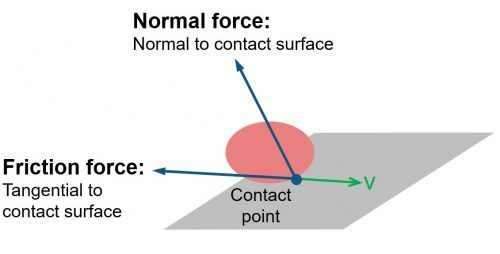Normal forces can be modeled in several ways, including

• Impulse-based: This is a one-time event that occurs when two objects collide. Before collision, each object has a certain momentum (mass * velocity, or its rotational equivalent). After the collision, the magnitude and direction of each object’s momentum will change based on the type of collision; for example, elastic vs. inelastic. This approach works best in models where the objects do not frequently move in and out of contact.
• Force-based: Normal forces are applied to objects based on a force law. A common approach is to apply a penalty force; for example, treating contact surfaces as springs and dampers whose coefficients approximate real world behavior. Penalty forces allow objects to overlap, which can approximate the deformation (or “squishing”) of these objects when they collide. Unlike the impulse-based approach, a force-based collision may take multiple simulation time steps to reach steady-state.
• Motion constraint: Here, we assume that objects are always in contact, so we do not have to worry about collision dynamics. For example, if we assume that a wheel is always on the ground, we do not need to model the normal forces; we only need a friction model to relate the rotation of the wheel with its linear motion.

Friction forces typically consist of a force law with two distinct regions.

• Static friction represents the initial force needed by an object at rest to begin sliding along its contact surface.
• Kinetic friction represents the resistive force of an object when it is moving along its contact surface.

Friction force curves can be represented using equations, or by directly using the curve data in a lookup table.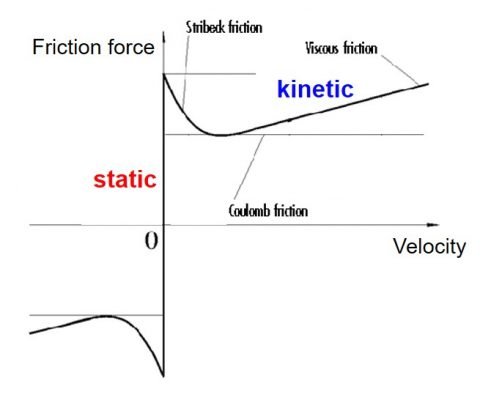## Contact with Simulink®

Simulink® lets you graphically implement equations. Mechanical system dynamics can be modeled using differential equations, which are commonly associated with with the Integrator block. This is because of Newton’s 2nd Law (Force = mass*acceleration). The acceleration can be integrated once to calculate velocity, and again to calculate position.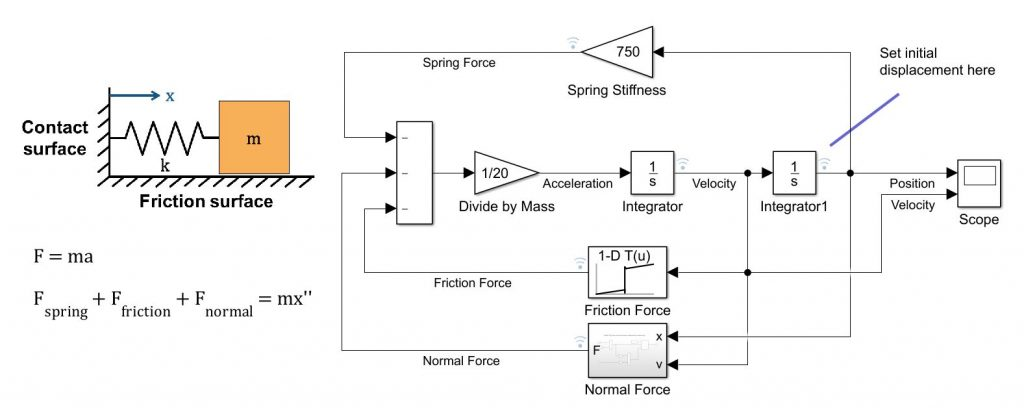To implement force-based contact and friction forces, you can represent the force laws using blocks for mathematical operations or by directly representing the friction curves as lookup tables. These forces can then be summed up and passed into the Integrator blocks as shown below.## 1D Contact with Simscape™

Simscape™ extends Simulink® for modeling 1D physical networks (electrical, fluid, mechanical, etc.) without deriving equations of motion. Every mechanical Simscape™ component is implemented by establishing a relationship between velocity and force/torque, so you can implement 1D force-based contact in Simscape™.

Simscape™ has built-in blocks such as the Translational Friction and Translational Hard Stop blocks that provide a good starting point for contact modeling. If you want to implement a different normal or friction force law, you can view the source code of Simscape™ blocks and customize their equations – or you can create your own component from scratch.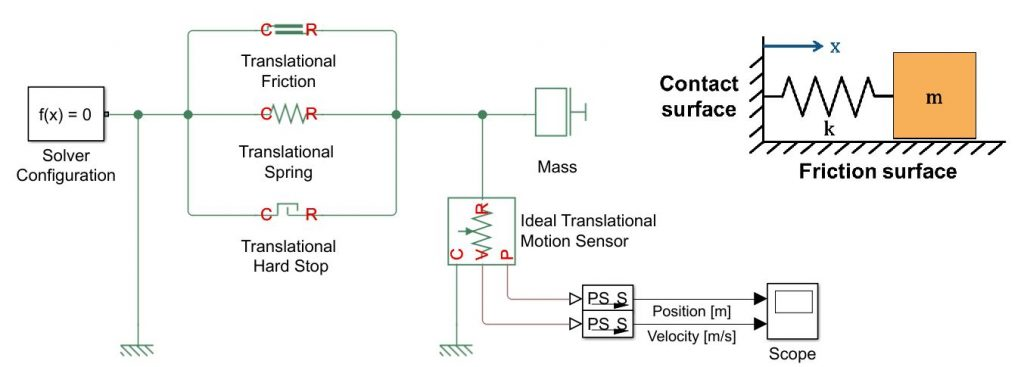## 3D Contact with Simscape Multibody™

You can use Simscape Multibody™ to model 3D rigid-body systems. There are two types of contact that you can implement with these models.

If you’re interfacing 1D contact into a larger 3D model, for example, actuator limits or friction, you can use the Simscape Multibody™ Multiphysics Library to help you connect an individual Revolute or Prismatic Joint block to the Hard Stop and Friction blocks described above.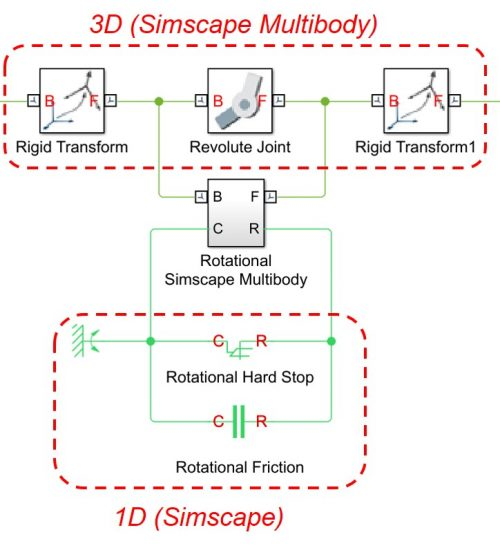Else, you can implement a more generic (2D or 3D) penalty force relationship by

1. Measuring the relative motion between two objects (Transform Sensor)
2. “Doing the math” with Simulink® blocks
3. Applying the resulting contact forces/torques to the objects in contact (External Force + Torque)

The Simscape Multibody™ Contact Forces Library already implements these forces for certain basic shapes, like spheres, planes, and tubes. I strongly recommend using this library a starting point, and if you need to implement something different you can customize these blocks.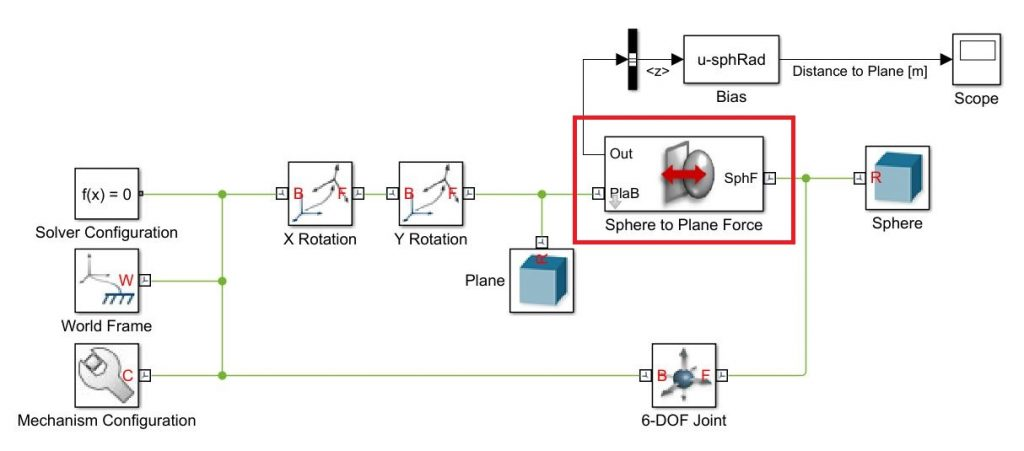## Conclusion

We just covered several ways to model mechanical contact in Simulink®, Simscape™, and Simscape Multibody™. To summarize,

• Simulink® is the most flexible tool and lets you implement impulse-based and force-based contact. However, you need to derive all the equations of motion.
• Simscape™ lets you model 1D mechanisms without deriving equations of motion, and contains basic blocks (which are customizable) to represent normal and friction contact forces.
• Simscape Multibody™ let you model 3D rigid body systems without deriving equations of motion.

For more information, watch the videos in this blog post. You can also download example models from the File Exchange.

Are you planning, or working on, simulations with mechanical contact? Do you have any comments about the approaches or libraries we shared in this post? We’d be interested in hearing from you.

– Sebastian

|

### Comments

To leave a comment, please click here to sign in to your MathWorks Account or create a new one.